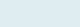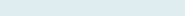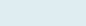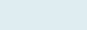# Date based on Week Number

To extract date and day from the week number and year. suppose you want to know the day (Monday, Tuesday, etc) and date based on any week number.

We have two columns, Year and Week. Our task is to know the date based on the given year and week number.

Formula to implement:

=DATE(B7,1,-2)-WEEKDAY(DATE(B7,1,3))+C7*7

Function applicable:

Date: The Excel DATE function returns a date value using year, month and date, sequentially.

Weekday: The Excel WEEKDAY function returns an integer between 1 and 7 the corresponds specific day of a week.

Formula Explanation (step by step)

Use shortcut F9 function key in Excel, tab clicks on evaluating the formula.

Let’s break the formula into multiple small steps to understand its application.

First formula: DATE(B7,1,-2)

Syntax: =DATE(year, month, day)

Step 1:Result: 29-12-2013

It returns date after subtracting 2 days from the given date. here we subtract 2 days you can subtract any days.

Second formula: =DATE(B7,1,-2)-WEEKDAY(DATE(B7,1,3))+C7*7

Syntax: =WEEKDAY(serial_number,[return_type])

Step 2:Result: 6

Now, It returns weekday (6) on the basis of given date (3), month (1) and year (2014).

Step 3:Result: 17-Febuarary-2014The formula will first subtract date “29-12-2013” from 6 it returns “23-12-2013” and then add date after multiplying C7*7 (8*7) gives 56. Now add 56 dates to 23-12-2013 it returns 8th week with the day (Monday) by multiplying 7 i.e. “17-Feb-2014”.

Remember: To know the exact day name multiply it with 7 with dates.